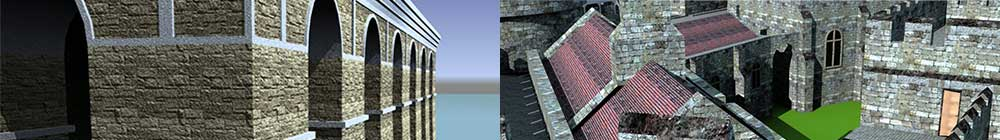SketchUp
Dynamic
Components

# 7. Dynamic copies

Previous lessons have looked at simple dynamics such as changing a single attribute but this lesson will tackle something more complicated and which can sometimes take a while to get your head around – dynamic copies.

As an example, imagine a ladder with 10 rungs spaced 50 cm apart. If you double the length of the ladder, you don't want 10 rungs spaced a metre apart, you want 20 rungs spaced 50 cm apart. Similar needs come with sets of stairs, fences, furniture with multiple drawers.

For this tutorial, we shall again keep things simple so you can understand the principles without getting confused by details. Start by creating a block 100 x 50 x 200 on each side, group it and name it "Wall". Then create a step 50 x 10 x 30, make it a component (because it will be repeated, unlike the wall), and name it "Step". Position the step at the bottom edge of the block (see below), then select both and make it in to a component "DC4".

Notice that we have only made one rung. All the others will be handled and duplicated automatically by formulas but, before we do that, we shall add a couple of custom attributes. Under the DC4 component, add two custom attributes: "TotalHeight" and "Spacing". The first determines the total height of the component so enter "50" for the moment. Then, under the Wall element, add the attribute LenZ and enter into its box the formula:

`=DC4!totalHeight`

The height of the Wall is now linked to TotalHeight so we can change the height of the subcomponent Wall from the main DC4 component. The Spacing attribute determines how far apart the steps are so enter 50 at the moment (so we have one step per 50 cm of wall height).

If we now enter 100 into TotalHeight, we should see that the wall gets higher but nothing else happens. We need to handle the duplication of the steps to allow for the extra height and this is determined by the attribute called "Copies" (under OnClick in the popup menu). So add the Copies attribute to the Step element (since we are making copies of the Step and enter the formula:

`=DC4!totalHeight/DC4!Spacing`

This means that the height of the wall is divided by Spacing to determine the number of total steps. If you try different values in TotalHeight, you should see the value in Copies change:

TotalHeight Formula Copies
50 50/50 = 1
100 100/50 = 2
150 150/50 = 3

So the number of steps is increasing with the height of the Wall. Wait, you protest, I can only see one Step! Well there really are the same number of Steps to match the Copies value but they are all in the same location as the original Step so you can't see them. What we need to do now is change the Y and Z positions of the copies of the Step so that they are positioned separately. This is handled by a new, read-only attribute which is not normally visible called COPY. This holds the number of each copy so the original Step is numbered 0, the next 1, then 2, and so on. If you view the X, Y and Z positions of the Step, you see that it shows X=100, Y=0 and Z=0. If we start with the vertical position of each Step, we already have the Spacing so we simply set the Z position to:

`=DC4!Spacing*COPY`

The results would be:

COPY No. Formula Z Position
0 50*0 = 0
1 50*1 = 50
2 50*2 = 100

etc.

After entering this formula, you should see the steps suddenly appear next to the wall.

You can change the horizontal position as well by adding the same formula to the Y position. Now, as you change the TotalHeight, you will see Steps magically appear or disappear.

if you double-click and open the component after these copies have been made and select one of the them, you will see the COPY number in the attributes (below)

If your component isn't working, here are the settings below:

Obviously the positioning isn't quite right and here comes the mathematical bit. You need to adjust the formulas to space things correctly, maybe adding on an offset or allowing for the thickness of the step. One of the best ways to go about this is a sort of reverse engineering – draw the final component on paper, working out what the positions of each step would be and work out a formula which would produce that. A spreadsheet comes in very useful here.

The final formulas which space the Steps correctly are:

`Y position = (200-30)/Copies*Copy`
`Z position = (DC4!totalHeight-10)/Copies*Copy`

As before, the component, DC4, is available on 3D Warehouse at